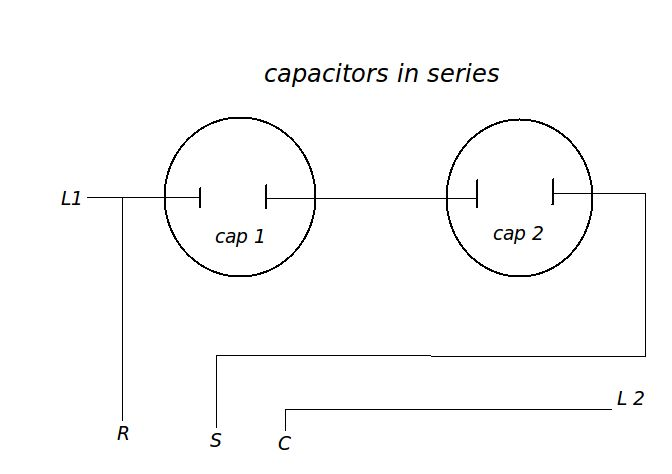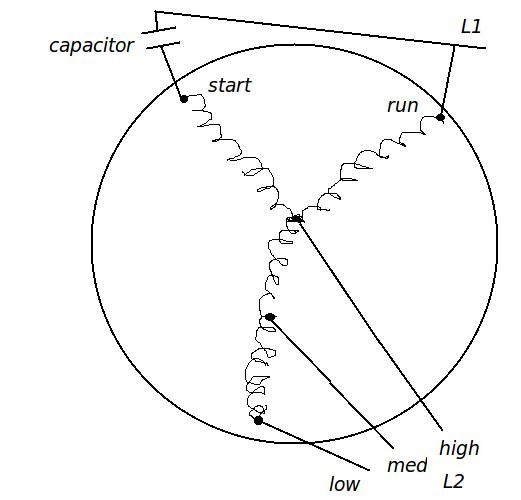Motor and Capacitor Page

Testing a capacitor while running in the circuit
V= Voltage measured between run & start terminals
A = Amps measured at start wire going to compressor
Formula: uf = 2652 * Amps / Volts
Voltage
Amps
capacitors in series calculator

Capacitors in series can never be bigger than the smallest size in the series so a 5uf & 85uf will be smaller than 5uf.

Two of the same size capacitors in series equals 1/2 of the rating of one. So two 10uf caps = 5uf

 formula: uf= 1/ (1/cap1 + 1/cap2 ...) Cap 1: Cap 2: Cap 3:Enter any two numbers to get the third number
 Capacitor 1: Capacitor 2: Series uf=

Motor info: If you look at the diagram of a multi-speed motor, the speed taps actually all connect to the common motor winding.